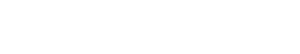# Algorithm

An algorithm is a set of instructions or rules that are followed in order to solve a problem or accomplish a task. In the text analytics industry, algorithms are used to process and analyze text data in order to extract valuable insights and information.

Algorithms can be simple or complex, but they all follow a set of steps or rules in order to achieve a desired outcome. For example, a simple algorithm might be used to sort a list of names alphabetically. A more complex algorithm might be used to analyze a large dataset in order to detect patterns or trends.

Text analytics algorithms are often used to perform tasks such as identifying key phrases or topics, sentiment analysis, and entity extraction. These algorithms can be proprietary or open source , and they can be customized or configurable to meet the specific needs of an organization.

## Techniques Used in Algorithm :

• tokenization
• stop word removal
• stemming and lemmatization
• part-of-speech tagging
• named entity recognition
• sentiment analysis
• topic modeling

## Algorithm may also refer to the following:

• A mathematical formula or set of rules used to solve a problem
• A step-by-step process for solving a problem
• A set of instructions for a computer to follow
• A set of rules used in logic or decision making
• A sequence of operations that are performed in order to solve a problem

## Algorithm vs. Heuristic

It is important to note that there is a difference between an algorithm and a heuristic. An algorithm is a set of rules or instructions that always produces the same result, given the same inputs. A heuristic is a rule of thumb or an approximate method that may not always produce the same result, but is typically faster than using an algorithm.

For example, a sorting algorithm will always produce the same sorted list of items, regardless of who is using it or how many times it is used. A heuristic for sorting a list might be to simply put the items in alphabetical order, which would be faster than using a sorting algorithm, but would not always produce the same results.

## Algorithm vs. Machine Learning

It is also important to note that there is a difference between an algorithm and machine learning. An algorithm is a set of rules or instructions that are followed in order to solve a problem or accomplish a task. Machine learning is a subset of artificial intelligence that focuses on the ability of computers to learn from data and improve their performance over time without being explicitly programmed.

Machine learning algorithms are often used for tasks such as image recognition, spam detection, and fraud detection. These algorithms can be trained on large datasets in order to learn from past data and make predictions about future data.Unlock the power of actionable insights with AI-based natural language processing.